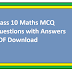# Class 10 Maths MCQ Questions with Answers PDF: Ace Your Exams!

Introduction: Class 10 mathematics can be a challenging subject, but with the right practice and resources, you can excel in it. Multiple-choice questions (MCQs) are a valuable tool for assessing your understanding of various mathematical concepts. To help you prepare effectively, we've compiled a set of Class 10 Maths MCQ questions with answers in a convenient PDF format. In this article, we'll provide you with a sneak peek of the topics covered and the benefits of using MCQs for your exam preparation.

### Benefits of MCQs for Class 10 Maths Preparation:

1. Focused Learning: MCQs are designed to test specific concepts, helping you pinpoint areas where you need improvement.

2. Quick Assessment: MCQs are time-efficient and allow you to evaluate your knowledge of multiple topics in a shorter time frame.

3. Variety of Questions: Our PDF includes a wide range of MCQs covering different chapters, ensuring comprehensive practice.

4. Immediate Feedback: You can check your answers instantly, identifying any mistakes and learning from them.

### Topics Covered in the PDF:

1. Real Numbers: MCQs related to properties of real numbers, Euclid's division lemma, and the Fundamental Theorem of Arithmetic.

2. Polynomials: Questions on factors and zeros of polynomials, factorization techniques, and algebraic identities.

3. Linear Equations: MCQs covering linear equations in two variables, their graphical representation, and applications.

4. Quadratic Equations: Questions related to the discriminant, nature of roots, and solving quadratic equations.

5. Arithmetic Progressions: MCQs on finding the nth term, sum of n terms, and other properties of arithmetic progressions.

6. Triangles: Questions on congruence of triangles, the Pythagorean theorem, and properties of triangles.

7. Coordinate Geometry: MCQs focusing on the distance formula, section formula, and graphical representation of lines and curves.

8. Introduction to Trigonometry: Questions related to trigonometric ratios, complementary angles, and trigonometric identities.

9. Statistics and Probability: MCQs covering topics like mean, median, mode, and probability.

10. Mensuration: Questions on areas and volumes of various geometric shapes.

12. Circles: Questions on theorems related to circles, chords, and tangents.

### How to Use the PDF:

2. Print or Use Digitally: You can either print the PDF or use it digitally on your computer or tablet.

3. Practice Regularly: Set aside dedicated study time to work through the MCQs. Try to solve them without looking at the answers first.

Class 10 Maths MCQ Questions with Answers PDF DownloadReviewed by SSC NOTES on September 01, 2023 Rating: 5